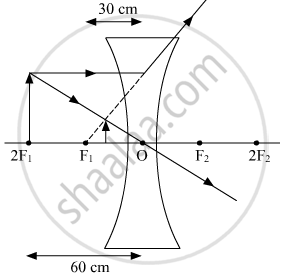# An Object is Placed at a Distance of 60 Cm from a Concave Lens of Focal Length 30 Cm. (I) Use the Lens Formula to Find the Distance of the Image from the Lens. - Science

#### Question

An object is placed at a distance of 60 cm from a concave lens of focal length 30 cm.
(i) Use the lens formula to find the distance of the image from the lens.
(ii) List four characteristics of the image (nature, position, size, erect/inverted) formed by the lens in this case.
(iii) Draw a ray diagram to justify your answer of the part (ii).

#### Solution

We have,

(i) Object distance, u= —60 cm Focal length of the concave lens, f= —30 cm Using lens formula,
1/"v"-1/"u" = 1/"f"

1/"v" - 1/((-60)) =1/((-30))

1/"v"=(-1)/30-1/60

1/"v"=(-3)/60

v = -20 cm
The image will be formed at a distance of 20 cm in front of the lens.

(ii) Nature of the image is virtual. The position of the image is between F1 and optical center 0. Size of the image is diminished. The image is Erect.

(iii)Is there an error in this question or solution?